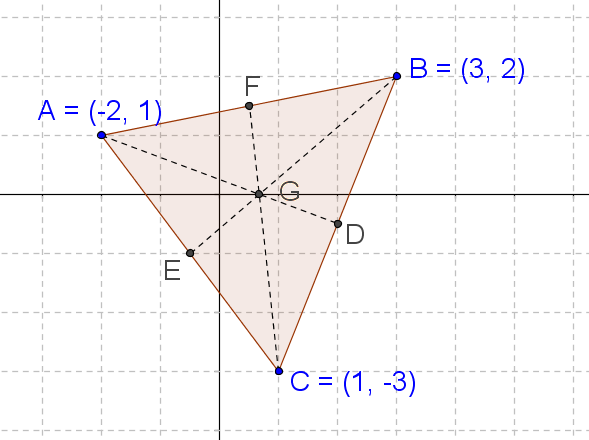# General Equation of a Line

Go back to  'Coordinate Geometry'

By now, it should be evident that the general equation of a line has an x term, a y term and a constant term, so that we can write it as follows:

$ax + by + c = 0$

We have also seen this form in the chapter on two-variable linear equations. Note that at least one of a and b must be non-zero.

We can convert any form of the line’s equation into the general form. For example, consider a line which passes through $$\left( {1,3} \right)$$ and has the slope $$- 2$$. Using the point-slope form, the equation of this line is:

$\begin{array}{l}y - 3 = - 2\left( {x - 1} \right)\\ \Rightarrow \,\,\,y - 3 = - 2x + 2\end{array}$

We can rearrange this and write this in the general form as follows:

$2x + y - 5 = 0$

The general form of a line’s equation is also known as the standard form.

Example 1: Write the equation of the line passing through the points $$\left( { - 1,3} \right)$$ and $$\left( {2, - 3} \right)$$ in standard form.

Solution: Using the two-point form, the equation of the line is

\begin{align}&\frac{{y - {y_1}}}{{x - {x_1}}} = \frac{{{y_2} - {y_1}}}{{{x_2} - {x_1}}}\\&\Rightarrow \,\,\,\frac{{y - 3}}{{x - \left( { - 1} \right)}} = \frac{{ - 3 - 3}}{{2 - \left( { - 1} \right)}}\\&\Rightarrow \,\,\,\frac{{y - 3}}{{x + 1}} = \frac{{ - 6}}{3} = - 2\\&\Rightarrow \,\,\,y - 3 = - 2x - 2\\&\Rightarrow \,\,\,2x + y - 1 = 0\end{align}

The last equation is the required equation in standard form.

Example 2: The equation of a line is $$ax + by + c = 0$$ . Find the slope, the x-intercept and the y-intercept of the line.

Solution: To find the slope, we rearrange the given equation to the slope-intercept form:

\begin{align}&by = - ax - c\\&\Rightarrow \,\,\,y = \left( { - \frac{a}{b}} \right)x + \left( { - \frac{c}{b}} \right)\end{align}

Thus, the slope of the line is $$m = - \frac{a}{b}$$ , while the y-intercept is $$- \frac{c}{b}$$. To find the x-intercept, we substitute $$y = 0$$ in the equation of the line:

\begin{align}&ax + b\left( 0 \right) + c = 0\\&\Rightarrow \,\,\,ax + c = 0\\&\Rightarrow \,\,\,x = - \frac{c}{a}\end{align}

Thus, the x-intercept is $$- \frac{c}{a}$$.

Example 3: A triangle has the vertices $$A\left( { - 2,1} \right)$$, $$B\left( {3,2} \right)$$, and $$C\left( {1, - 3} \right)$$. Find the coordinates of the centroid G, and the equations of the three medians of the triangle.

Solution: Consider the following figure:The coordinates of D, E and F are:

\begin{align}&D \equiv \left( {\frac{{3 + 1}}{2},\frac{{2 + \left( { - 3} \right)}}{2}} \right) = \left( {2, - \frac{1}{2}} \right)\\&E \equiv \left( {\frac{{1 + \left( { - 2} \right)}}{2},\frac{{\left( { - 3} \right) + 1}}{2}} \right) = \left( { - \frac{1}{2}, - 1} \right)\\&F \equiv \left( {\frac{{ - 2 + 3}}{2},\frac{{1 + 2}}{2}} \right) = \left( {\frac{1}{2},\frac{3}{2}} \right)\end{align}

The coordinates of the centroid G are:

$G \equiv \left( {\frac{{ - 2 + 3 + 1}}{3},\frac{{1 + 2 + \left( { - 3} \right)}}{3}} \right) = \left( {\frac{2}{3},0} \right)$

Now, using the two-point form, we determine the equation of AD:

\begin{align}&\frac{{y - {y_1}}}{{x - {x_1}}} = \frac{{{y_2} - {y_1}}}{{{x_2} - {x_1}}}\\&\Rightarrow \,\,\,\frac{{y - 1}}{{x - \left( { - 2} \right)}} = \frac{{ - \frac{1}{2} - 1}}{{2 - \left( { - 2} \right)}}\\&\Rightarrow \,\,\,\frac{{y - 1}}{{x + 2}} = - \frac{3}{8}\\&\Rightarrow \,\,\,8y - 8 = - 3x - 6\\&\Rightarrow \,\,\,3x + 8y - 2 = 0\end{align}

Similarly, the equations of BE and CF can be determined:

$\begin{array}{l}BE:\,\,\,6x - 7y - 4 = 0\\CF:\,\,\,9x + y - 6 = 0\end{array}$